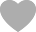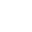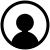DiscoverArtificial IntelligenceClaim Ownership

# #box-pro-ellipsis-157943881075413{-webkit-line-clamp:2;}Artificial Intelligence

Subscribed: 476Played: 893
Share

Description

In these lectures, Prof. Patrick Winston introduces the 6.034 material from a conceptual, big-picture perspective. Topics include reasoning, search, constraints, learning, representations, architectures, and probabilistic inference. In these mega-recitations, teaching assistant Mark Seifter works through problems from previous exams in a lecture-style setting. Students are asked to participate, and emphasis is placed on being able to work the algorithms by hand.
31 Episodes
Reverse

Lecture 12b: Deep Neural Nets

2016-03-0400:49:051

In this lecture, Prof. Winston discusses BLANK and modern breakthroughs in neural net research.
In this video, Prof. Winston introduces neural nets and back propagation.
This mega-recitation covers Problem 1 from Quiz 2, Fall 2007. We start with a minimax search of the game tree, and then work an example using alpha-beta pruning. We also discuss static evaluation and progressive deepening (Problem 1-C, Fall 2008 Quiz 2).
This mega-recitation covers a question from the Fall 2007 final exam, in which we teach a robot how to identify a table lamp. Given a starting model, we identify a heuristic and adjust the model for each example; examples can be hits or near misses.
We start by discussing what a support vector is, using two-dimensional graphs as an example. We work Problem 1 of Quiz 4, Fall 2008: identifying support vectors, describing the classifier, and using a kernel function to project points into a new space.
This mega-recitation covers the boosting problem from Quiz 4, Fall 2009. We determine which classifiers to use, then perform three rounds of boosting, adjusting the weights in each round. This gives us an expression for the final classifier.
We begin by discussing neural net formulas, including the sigmoid and performance functions and their derivatives. We then work Problem 2 of Quiz 3, Fall 2008, which includes running one step of back propagation and matching neural nets with classifiers.
This mega-recitation covers Problem 2 from Quiz 1, Fall 2008. We start with depth-first search and breadth-first search, using a goal tree in each case. We then discuss branch and bound and A*, and why they give different answers in this problem.
In this mega-recitation, we cover Problem 1 from Quiz 1, Fall 2009. We begin with the rules and assertions, then spend most of our time on backward chaining and drawing the goal tree for Part A. We end with a brief discussion of forward chaining.
This lecture begins with a brief discussion of cross-modal coupling. Prof. Winston then reviews big ideas of the course, suggests possible next courses, and demonstrates how a story can be understood from multiple points of view at a conceptual level.
We begin this lecture with basic probability concepts, and then discuss belief nets, which capture causal relationships between events and allow us to specify the model more simply. We can then use the chain rule to calculate the joint probability table.
We begin with a review of inference nets, then discuss how to use experimental data to develop a model, which can be used to perform simulations. If we have two competing models, we can use Bayes' rule to determine which is more likely to be accurate.
In this lecture, we consider cognitive architectures, including General Problem Solver, SOAR, Emotion Machine, Subsumption, and Genesis. Each is based on a different hypothesis about human intelligence, such as the importance of language and stories.
Can multiple weak classifiers be used to make a strong one? We examine the boosting algorithm, which adjusts the weight of each classifier, and work through the math. We end with how boosting doesn't seem to overfit, and mention some applications.
In this lecture, we consider the nature of human intelligence, including our ability to tell and understand stories. We discuss the most useful elements of our inner language: classification, transitions, trajectories, and story sequences.
In this lecture, we explore support vector machines in some mathematical detail. We use Lagrange multipliers to maximize the width of the street given certain constraints. If needed, we transform vectors into another space, using a kernel function.
To determine whether three blocks form an arch, we use a model which evolves through examples and near misses; this is an example of one-shot learning. We also discuss other aspects of how students learn, and how to package your ideas better.
This lecture explores genetic algorithms at a conceptual level. We consider three approaches to how a population evolves towards desirable traits, ending with ranks of both fitness and diversity. We briefly discuss how this space is rich with solutions.
Why do "cats" and "dogs" end with different plural sounds, and how do we learn this? We can represent this problem in terms of distinctive features, and then generalize. We end this lecture with a brief discussion of how to approach AI problems.
This lecture begins with a high-level view of learning, then covers nearest neighbors using several graphical examples. We then discuss how to learn motor skills such as bouncing a tennis ball, and consider the effects of sleep deprivation.